# Statistics Learning - (Error|misclassification) Rate - false (positives|negatives)

The error rate is a prediction error metrics for a binary classification problem.

The error rate metrics for a two-class classification problem are calculated with the help of a Confusion Matrix.

The below confusion matrix shows the results for a two-class classification problem where the target can take the value:

• Positive
• Or Negative

True = Truth = Good Predictions

## Rate

False rate are not desired while true rate are.

For instance, in a spam application, a false negative will deliver a spam in your inbox and a false positive will deliver legitimate mail to the junk folder.

### False

#### False Positive

False Positive (also known as false alarm) are predictions that should be false but were predicted as true

False positive rate: The fraction of negative examples (No, False, -) that are classified as positive (Yes, True, +) $$\begin{array}{rrl} \text{False positive rate} & = & \frac{\text{False Positive}}{\text{False Positive} + \text{True Negative}} \\ & = & \frac{c}{c + d} \\ & = & 1- \text{accuracy of the other class} \end{array}$$

#### False Negative

False Negative: Predictions that should be true but were predicted as false

False negative rate: The fraction of positive examples that are classified as negative $$\begin{array}{rrc} \text{False negative rate} & = & \frac{b}{a + b} \end{array}$$

### True

#### True Positive

True Positive: positive instances that are correctly assigned to the positive class

The TP rate is the same as the accuracy for the first class.

True Positive rate: The fraction of positive target that are classified as positive $$\begin{array}{rrl} \text{True positive rate} & = & \frac{\text{True Positive}}{\text{True Positive} + \text{False Negative}} \\ & = & \frac{a}{a + b} \\ & = & \text{Accuracy on the first class} \end{array}$$

## How to decrease it

In a probability regression classification for a two-class problem, the threshold is normally on 50%. Above the threshold, it will be 1 and below it will be 0.

$$\hat{Pr}(Y = Yes|X ) \geq threshold$$

By changing this classification probability threshold, you can improve this error rate.

In a regression classification, algorithm, you capture the probability threshold changes in an ROC curve.

For instance: (For a two class, the threshold is 0.5)

The Total Error is a weighted average of the False Positive Rate and False Negative Rate. The weights are determined by the Prior Probabilities of Positive and Negative Responses. Positive responses are so uncommon that the False Negatives makes up only a small portion of the Total error therefore Total Error keep going down even though the False Negative Rate increases.

## Others Metrics

### Sensitivity / Specificity

#### Sensitivity

$$\begin{array}{rrl} Sensitivity & = & \frac{a}{a+b} \\ \end{array}$$

#### Specificity

$$\begin{array}{rrl} 1-Specificity & = & 1-\frac{d}{c+d} \end{array}$$

### Lift , Precision, Recall

Lift , Precision, Recall are others Accuracy Metrics

$$\begin{array}{rrl} Lift & = & \frac\text{% positives > threshold}}\text{% data set > threshold}} & = & \frac{a/(a +b)}}{(a + c)/(a + b + c + d)}}\\ Precision & = & \fraca}a+c}\\ Recall & = & \fraca}a+b}\\ \end{array}$$

Recommended Pages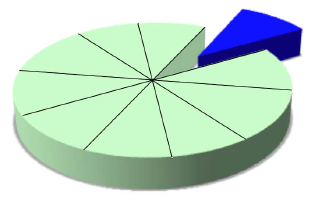(Statistics|Data Mining) - (K-Fold) Cross-validation (rotation estimation)

Cross-validation, sometimes called rotation estimation is a resampling validation technique for assessing how the results of a statistical analysis will generalize to an independent new data set. This...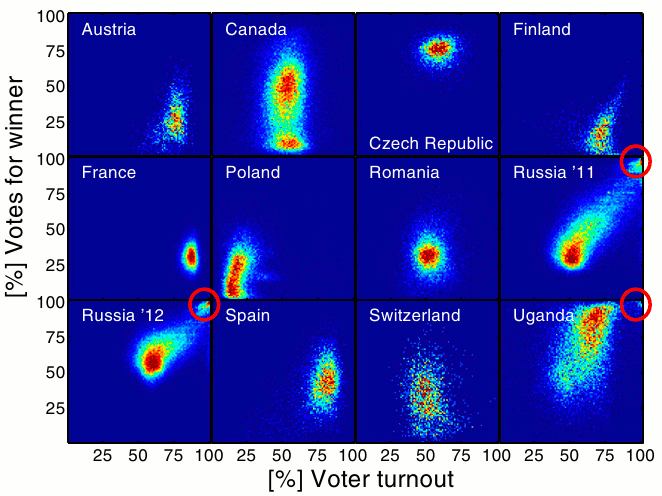Data Mining - (Anomaly|outlier) Detection

The goal of anomaly detection is to identify unusual or suspicious cases based on deviation from the norm within data that is seemingly homogeneous. Anomaly detection is an important tool: in data...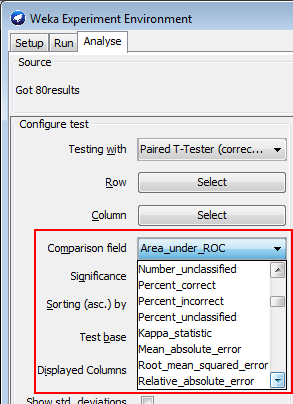Data Mining - (Parameters | Model) (Accuracy | Precision | Fit | Performance) Metrics

Accuracy is a evaluation metrics on how a model perform. rare event detection Hypothesis testing: t-statistic and p-value. The p value and t statistic measure how strong is the...Machine Learning - Confusion Matrix

If a classification system has been trained a confusion matrix will summarize the results (ie the error rate (false|true) (positive|negative) for a binary classification). overfitting The main diagonal...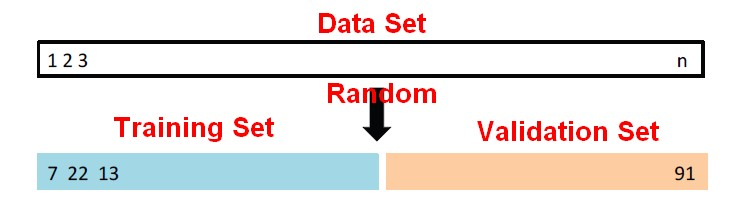Statistical Learning - Two-fold validation

Two-fold validation is a resampling method. It randomly divides the available set of samples into two parts: a training set and a validation or hold-out set. The model is fit on the training set,...Statistics - (Base rate fallacy|Bonferroni's principle)

Every accurate (model|test) can be useless as detection tools if the studied case is sufficiently rare among the general population. The data model will produce too many false positives or false negatives....Statistics - (Threshold|Cut-off) of binary classification

The Threshold or Cut-off represents in a binary classification the probability that the prediction is true. It represents the tradeoff between false positives and false negatives. Normally, the cut-off...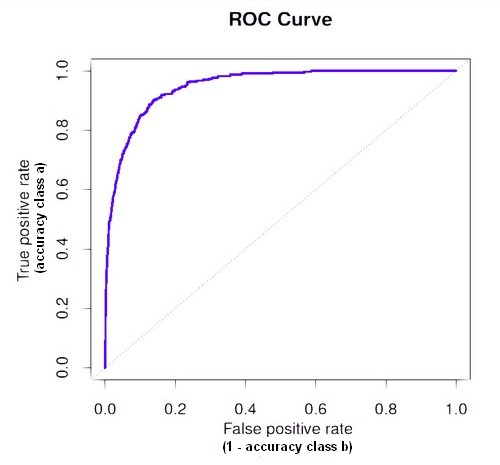Statistics - ROC Plot and Area under the curve (AUC)

The Area Under Curve (AUC) metric measures the performance of a binary classification. In a regression classification for a two-class problem using a probability algorithm, you will capture the probability...Statistics Learning - Prediction Error (Training versus Test)

The Prediction Error tries to represent the noise through the concept of training error versus test error. We fit our model to the training set. We take our model, and then we apply it to new data that...Viz - Control Chart (Shewhart)

The purpose of control charts is to allow simple detection of events that are indicative of actual process change. Control charts attempt to differentiate “assignable” (“special”) sources of variation...